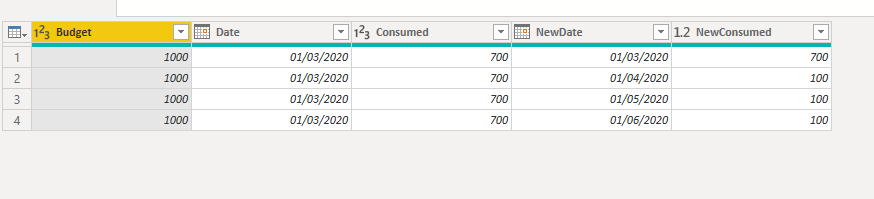cancel
Showing results for
Did you mean:Anonymous
Not applicable

## distribution of a budget in remaining Months

Hi everyone,

I have a budget and would like to distribut it in remaiming month of the year. for ex;

Budget =1000 for a year

actual till end of March = 700

remaining = 1000-700=300

remaining month to end of year = 3 month

so each month has = 100 (April =100, May=100 and Jun 100)

my formula is like below

column called divider

= Table.AddColumn(#"Removed Columns", "Divider", each if Date.Month(DateTime.LocalNow()) = 1 then 5 else if Date.Month(DateTime.LocalNow()) = 2 then 4 else if Date.Month(DateTime.LocalNow()) = 3 then 3 else if Date.Month(DateTime.LocalNow()) = 4 then 2 else if Date.Month(DateTime.LocalNow()) = 5 then 1 else if Date.Month(DateTime.LocalNow()) = 6 then 1 else if Date.Month(DateTime.LocalNow()) = 7 then 11 else if Date.Month(DateTime.LocalNow()) = 8 then 10 else if Date.Month(DateTime.LocalNow()) = 9 then 9 else if Date.Month(DateTime.LocalNow()) = 10 then 8 else if Date.Month(DateTime.LocalNow()) = 11 then 7 else if Date.Month(DateTime.LocalNow()) = 12 then 6 else null)

second column for April

Table.AddColumn(#"Added Custom9", "Apr", each if Date.Month(DateTime.LocalNow())=4 then 0 else if Date.Month(DateTime.LocalNow())<4 then 0 else [Cost To Complete]/[Divider])

* Cost To Complete = Total Budget - Actual budget.

my problem is, the formula doesn't show the correct answer? does anyone has a btter idea to distibute the remaining budget in remaining month ?

Thanks for every one

1 ACCEPTED SOLUTIONCommunity Champion

Hi @Anonymous ,

Try this m code:

let
Source = Table.FromRows(Json.Document(Binary.Decompress(Binary.FromText("i45WMjQwMFDSUTIw1Dcw1jcyMAJxzIFCsTrRSqZwKXOYlBFIKhYA", BinaryEncoding.Base64), Compression.Deflate)), let _t = ((type text) meta [Serialized.Text = true]) in type table [Budget = _t, Date = _t, Consumed = _t]),
#"Changed Type" = Table.TransformColumnTypes(Source,{{"Budget", Int64.Type}, {"Date", type date}, {"Consumed", Int64.Type}}),
_nMonths = if Date.Month([Date]) <= 6 then
6 - Date.Month([Date])
else
(12 - Date.Month([Date])) + 6,
_endDate = Date.AddMonths(_startDate, _nMonths + 1)
in
List.Select(List.Dates(_startDate, Duration.Days(_endDate - _startDate), #duration(1,0,0,0)), each Date.StartOfMonth(_) = _)),
#"Expanded Custom" = Table.ExpandListColumn(#"Added Custom", "Custom"),
#"Changed Type1" = Table.TransformColumnTypes(#"Expanded Custom",{{"Custom", type date}}),
#"Added Custom1" = Table.AddColumn(#"Changed Type1", "Custom.1", each if [Date] = [Custom] then [Consumed]
else
let _date = [Date] in
([Budget] - [Consumed]) / (Table.RowCount(Table.SelectRows(#"Expanded Custom", each [Date] = _date)) - 1)),
#"Renamed Columns" = Table.RenameColumns(#"Added Custom1",{{"Custom", "NewDate"}, {"Custom.1", "NewConsumed"}}),
#"Changed Type2" = Table.TransformColumnTypes(#"Renamed Columns",{{"NewConsumed", type number}})
in
#"Changed Type2"Community Champion

Hi @Anonymous ,

Try this m code:

let
Source = Table.FromRows(Json.Document(Binary.Decompress(Binary.FromText("i45WMjQwMFDSUTIw1Dcw1jcyMAJxzIFCsTrRSqZwKXOYlBFIKhYA", BinaryEncoding.Base64), Compression.Deflate)), let _t = ((type text) meta [Serialized.Text = true]) in type table [Budget = _t, Date = _t, Consumed = _t]),
#"Changed Type" = Table.TransformColumnTypes(Source,{{"Budget", Int64.Type}, {"Date", type date}, {"Consumed", Int64.Type}}),
_nMonths = if Date.Month([Date]) <= 6 then
6 - Date.Month([Date])
else
(12 - Date.Month([Date])) + 6,
_endDate = Date.AddMonths(_startDate, _nMonths + 1)
in
List.Select(List.Dates(_startDate, Duration.Days(_endDate - _startDate), #duration(1,0,0,0)), each Date.StartOfMonth(_) = _)),
#"Expanded Custom" = Table.ExpandListColumn(#"Added Custom", "Custom"),
#"Changed Type1" = Table.TransformColumnTypes(#"Expanded Custom",{{"Custom", type date}}),
#"Added Custom1" = Table.AddColumn(#"Changed Type1", "Custom.1", each if [Date] = [Custom] then [Consumed]
else
let _date = [Date] in
([Budget] - [Consumed]) / (Table.RowCount(Table.SelectRows(#"Expanded Custom", each [Date] = _date)) - 1)),
#"Renamed Columns" = Table.RenameColumns(#"Added Custom1",{{"Custom", "NewDate"}, {"Custom.1", "NewConsumed"}}),
#"Changed Type2" = Table.TransformColumnTypes(#"Renamed Columns",{{"NewConsumed", type number}})
in
#"Changed Type2"